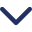# What is CAGR - Full form, Meaning, Formula and Calculator

Published On:
August 23, 2023
Updated On:
June 25, 2023CAGR or Compound Annual Growth Rate means measuring an investment's average annual growth rate over a specific period, assuming that the growth rate is constant throughout that period. Various metrics and indicators provide valuable insights into the performance and growth potential of different assets, businesses, and investment portfolios in finance and investing.

One such important metric is the Compound Annual Growth Rate (CAGR), a powerful tool used to analyze the growth rate of an investment over a specific period. This page explores the concept of CAGR, its meaning, formula, and how it can be calculated and interpreted.

## Meaning of CAGR:

The Compound Annual Growth Rate (CAGR) helps investors evaluate an investment's average annual growth rate over a specific period, assuming the growth rate is constant.

Unlike other measures, such as moderate or simple yearly growth rates, CAGR considers growth's compounding effect, making it a more accurate representation of long-term performance.

## Calculation of CAGR:

To calculate CAGR, you need the beginning, ending, and number of years for which the growth rate is calculated. The formula for CAGR is as follows:

CAGR = (Ending Value / Beginning Value)^(1 / Number of Years) - 1

For example, if you invested \$10,000 in stock and after five years, it grew to \$15,000, the CAGR would be:

CAGR = (15,000 / 10,000)^(1 / 5) - 1 = 0.122 or 12.2%

## CAGR Formula

The CAGR formula provides a standardized measure of growth that allows investors to compare the performance of different investments or assets over the same period. It smooths out the volatility and return fluctuations, offering a more comprehensive long-term growth picture. The formula for calculating Compound Annual Growth Rate (CAGR) is as follows:

CAGR = (Ending Value / Beginning Value)^(1 / Number of Years) - 1

To calculate CAGR, you need the following information:

• Ending Value: The value of the investment or asset at the end of the specified period.
• Beginning Value: The value of the investment or asset at the beginning of the specified period.
• Number of Years: The investment duration or growth rate period

#### Here's an example to illustrate the calculation:

• Suppose you invested \$10,000 in a stock, which grew to \$15,000 after five years.
• Ending Value = \$15,000 Beginning Value = \$10,000 Number of Years = 5
• CAGR = (15,000 / 10,000)^(1 / 5) - 1
• Simplifying the calculation:
• CAGR = 1.5^(0.2) - 1

Using a calculator or performing the calculation manually, we find:

CAGR = 0.122 or 12.2%

Therefore, the CAGR for this investment over the five years is 12.2%. This means that, on average, the investment grew at 12.2% per year over that time frame.

## Benefits of Using CAGR:

Here are some of the benefits of using the CAGR metric.

• Accurate Performance Assessment: CAGR accounts for the compounding effect and provides a reliable measure of the investment's performance over time.
• Standardized Comparison: It  allows for easy and meaningful comparisons between different investments or asset classes, facilitating informed decision-making
• Future Projection: By analyzing the historical CAGR, investors can estimate potential future returns and assess the viability of an investment strategy.
• Long-term Perspective: It  focuses on long-term growth, providing a more stable and representative measure of an investment's performance.

## Limitations of CAGR:

While CAGR is a valuable tool for assessing long-term growth, it's essential to be aware of the metric’s limitations:

• Ignores Short-term Volatility: It smoothes out short-term fluctuations, which means it may not capture the volatility experienced by an investment during the analyzed period.
• Unrealistic Assumption of Constant Growth: CAGR assumes a constant growth rate, which may not align with the actual market conditions or the performance of the investment.

## Conclusion:

The Compound Annual Growth Rate (CAGR) is a powerful metric that provides investors with a standardized and accurate measure of an investment's growth rate over a specific period. It  offers a more comprehensive and reliable assessment of long-term performance by considering the compounding effect of growth.

While it has limitations, CAGR is a valuable tool for evaluating investment opportunities, making informed decisions, and projecting future returns. Incorporating CAGR analysis into your investment strategy can enhance your understanding of growth potential and improve investment outcomes.

#### What is CAGR?Compound Annual Growth Rate (CAGR) is a measure used to determine the annual growth rate of an investment or business over a specific period of time. It provides a single rate of return that represents the compounded growth rate over the entire period.

#### How is CAGR calculated?CAGR is calculated using the following formula: CAGR = (Ending Value / Beginning Value) ^ (1 / Number of Years) - 1

#### What does CAGR represent?CAGR represents the annual growth rate of an investment or business assuming that it grows at a constant rate over the specified period. It helps to normalize the returns and provide a standardized measure of growth.

## Stay in the know

Get the latest product and management insights.